Know more about the Repeaters Batch Know more about the Repeaters Batch

# Electrochemistry

## What Is Electrochemistry?

Electrochemistry is the subdiscipline of chemistry that deals with the study of the relationship between electrical energy and chemical changes. Chemical reactions that involve the input or generation of electric currents are called electrochemical reactions. Such reactions are broadly classified into two categories:

1. Production of chemical change by electrical energy i.e. the phenomenon of electrolysis
2. Conversion of chemical energy into electrical energy. i.e., the generation of electricity by spontaneous redox reactions.

Electricity can be produced when electrons move from one element to another in certain types of reactions (such as redox reactions). Typically, electrochemistry deals with the overall reactions when multiple redox reactions occur simultaneously, connected via some external electric current and a suitable electrolyte. In other words, electrochemistry is also concerned with chemical phenomena that involve charge separation (as seen commonly in liquids such as solutions). The dissociation of charge often involves charge transfer that occurs homogeneously or heterogeneously between different chemical species.

## Electrochemical Cell

A spontaneous chemical process is the one which can take place on its own and in such a process Gibbs free energy of a system decreases. In electrochemistry, spontaneous reaction (redox reaction) results in the conversion of chemical energy into electrical energy. The reverse process is also possible where a non-spontaneous chemical reaction occurs by supplying electricity. These interconversions are carried out in equipment called electrochemical cell.

## Types of Electrochemical Cell

Electrochemical cell are of two types: galvanic cells and electrolytic cells

### Galvanic Cell

The galvanic cell converts chemical energy into electrical energy i.e, electricity can be obtained with the help of redox reaction. The oxidation and reduction take place in two separate compartments. Each compartment consists of an electrolyte solution and metallic conductor which acts as an electrode. The compartment containing the electrode and the solution of the electrolyte is called half cells.

For example- Daniell cell is a galvanic cell in which zinc and copper are used for a redox reaction to take place.

Zn(s) + Cu+2(aq) → Zn+2 + Cu(s)

At anode (oxidation half)

Zn(s) →Zn+2 + 2e

At cathode( reduction half)

Cu+2(aq) + 2e → Cu(s)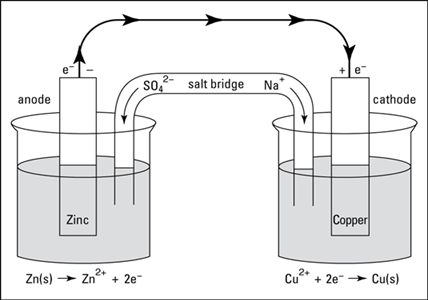Salt bridge: Salt bridge is usually an inverted U- tube filled with a concentrated solution of inert electrolytes. It is used to maintain the charge balance and to complete the circuit by allowing the flow of ions through it. It contains a gel in which inert electrolytes like KNO3 or K2SO4 are mixed. Through the salt bridge, negative ion flows towards the anode and positive ion flow to the cathode and the charge balance is maintained and cell keeps on functioning.

Electrode potential: In a galvanic cell, when two-electrode are dipped in their respective ion there is a tendency of one of the electrodes (anode) to undergo oxidation whereas the ion of the other electrode (cathode) has the tendency to gain an electron. This tendency of losing of electrons( oxidation) or gaining of electrons( reduction) is called electrode potential.

Standard electrode potential (E0): Standard electrode potential is defined as the electrode potential of an electrode relative to standard hydrogen electrode under standard conditions. The standard conditions taken are:

• 1 molar concentration of each ion in the solution.
• A Temperature of 298 K.
• 1 bar pressure.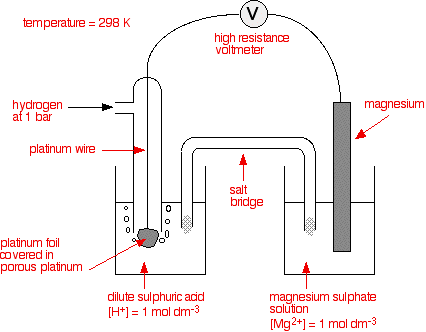Electrochemical Series

A series of the standard electrode has been established by measuring the potential of various electrodes versus standard hydrogen electrode(SHE). When the electrodes (metals and non-metals) in contact with their ions are arranged on the basis of the values of their standard reduction potential or standard oxidation potentials, the resulting series is called the electrochemical series.

Elements reduction reaction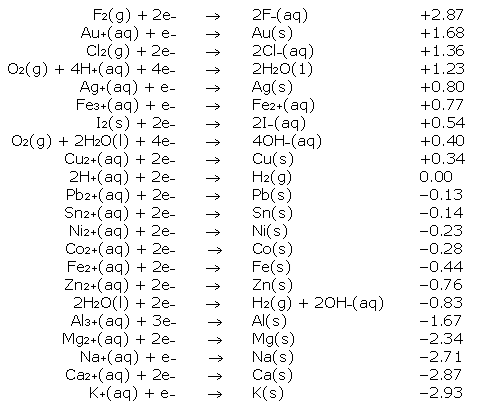Cell potential or emf of a cell: In the galvanic cell there are two half cell, the oxidation half-cell( anode) and the reduction half -cell( cathode).Due to the difference in the potentials of these half-cells, the electric current moves from the electrode of higher potential (cathode) to the electrode of lower potential( anode). The difference between the electrode potential of the two half cell is called cell potential or emf of a cell.

Eocell= ECathode– Eanode

ECathode= standard reduction potential of the cathode.

Eanode= standard reduction potential of the anode.

If Eocell is positive then the reaction is feasible.

If Eocell is negative then the reaction is not feasible.

Electrode and cell potentials- Nernst equation: The electrode potential and the emf of the cell depends upon the nature of the electrode, temperature and the activities( concentrations) of the ions in solution.

For the general electrochemical reaction of the type:

aA + bB → cC +dD

Ecell = Eocell – RT/ nF ln [C]c [D]d / [A]a [B]b

Ecell = Eocell – 2.0303 RT / nF log [C]c [D]d / [A]a [B]b

Ecell = Eocell – 0.0591 / n log [C]c [D]d / [A]a [B]b at 298K

### Electrolytic Cell

The electrolytic cell converts electrical energy to chemical energy. Here the electrodes are dipped in an electrolytic solution containing cations and anions. On supplying current the ions move towards electrodes of opposite polarity and simultaneous reduction and oxidation take place.

For example, in the electrolysis of molten sodium chloride, sodium chloride is melted (above 801oC), two electrodes are inserted into the melt, and an electric current is passed through the molten salt. The chemical reaction that takes place at the electrodes are:

● Sodium-ion migrates to the cathode, where sodium ion gains one electron and reduce to sodium metal.

Na+ + e→ Na

● Chloride ions migrate towards the anode where it loses one electron and gets oxidised to chlorine gas.

Cl→1/2 Cl2 + e

The overall reaction is the breakdown of sodium chloride into its elements

2NaCl→ 2Na(s) + Cl2(g)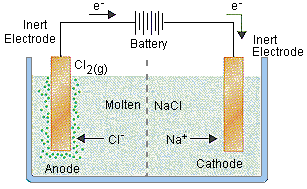Preferential discharge of ions: When there are more than one cations or anion the process of discharge becomes competitive in nature. For example, in the electrolysis of NaCl solution, apart from Na+ and Clions the solution of sodium chloride also contains H+ and OH ions due to ionisation of water. When the potential difference is applied between the two electrodes, Na+ and H+ ions move towards the cathode and Cl and OH ions move towards the anode. At cathode H+ ions get reduce in preference to giving hydrogen gas because hydrogen has a higher reduction potential than sodium. Similarly, at the anode, Cl ions are oxidised in preference to OHto give chlorine gas.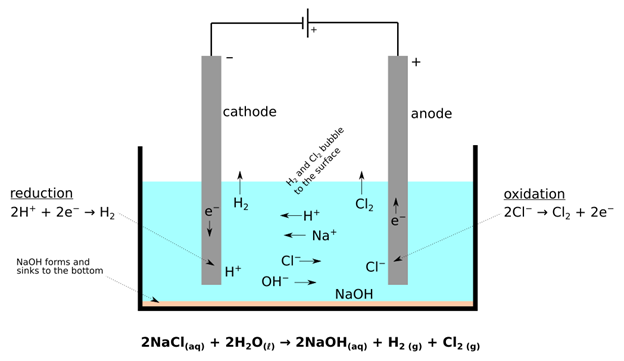The relationship between the quantity of electric charge passed through an electrolyte and the amount of substance deposited at the electrodes was given by Faraday in 1834, in the form of the law of electrolysis.

When an electric current is passed through an electrolyte, the amount of substance deposited is proportional to the quantity of electric charge passed through the electrolyte.

If W is the mass of the substance deposited by passing Q coulomb of charge then according to this law:

W ∝ Q

Now, Q = I ✕ t

W ∝ I ✕ t

W = z ✕ I ✕ t

Where Z is a constant known as electrochemical equivalent and is characteristic of a substance deposited.

Faraday’s constant(F) – It is the charge possessed by 1 mole of electrons and it is equal to 96500 coulombs(approx.). In terms of Faraday’s constant the number of gram equivalent of electrolyte discharged at an electrode is equal to the faraday’s passed

W = E X Q / 96500

When the same quantity of charge is passed through different electrolytes, then the mass of different substance deposited at the respective electrodes will be in the ratio of their equivalent masses.

Mathematically it is represented as;

W1 / W2 = Z1 / Z2

Where W1 and W2 are the weight of two substances which are deposited at their respective electrodes and Z1 and Z2 are their respective equivalent weight.

## Solved Problems

1. Given that,

Fe2+/ Fe = -0.44 v, Ni+2/ Ni = 0.25 v

Ag+ / Ag = 0.80 v, Cu+2/ Cu = 0.34 v

Which of the following reactions under standard condition will not take place in the specified direction.

Ni+2(aq) + Cu(s) → Ni(s) + Cu+2(aq)

Cu(s) + 2 Ag+(aq) → Cu+2(aq) + 2 Ag(s)

Cu(s) + 2H+(aq)→ Cu+2 + H2 (g)

Fe(s) + 2H+(aq)→ Fe+2 (aq) + 3 H2

Solution:

Option a and c

Ni+2(aq) + Cu(s) → Ni(s) + Cu+2(aq)

Eocell= 0.25 – 0.34

= -0.11v

Reaction is not feasible (negative Eocell)

Cu(s) + 2 Ag+(aq) → Cu+2(aq) + 2 Ag(s)

Eocell =0.80 – 0.34 = 0.46 v

Reaction is feasible( positive Eocell)

Cu(s) + 2H+(aq)→ Cu+2 + H2 (g)

Eocell = 0 – 0.34 = – 0.34 v

Reaction is not feasible ( negative Eocell)

Fe(s) + 2H+(aq) → Fe+2 (aq) + 3 H2

Eocell = 0 – (-0.44) = 0.44 v

Reaction is feasible( positive Eocell)

2. The emf of a cell corresponding to the reaction, Zn + 2H+ (aq) Zn+2 (0.1M) + H2(g) 1 atm is 0.30 v at 25 0 C. Write the half cell reactions and calculate the pH of the solution at the hydrogen electrode. ( E0cell = – 0.76 v )

Solution :

Eocell = 0 – (-0.76) = 0.76 v

Applying the Nernst equation,

Ecell = Eocell – 0.0591 / 2 log [zN+2][H2] / [H]+

0.30 = 0.76 – 0.0591 / 2 log 0.1 x 1 / [H+]2

log 0.1 / [H+]2 = 2 x 0.46 / 0.0591

log 0.1 – log [H+]2 = 15.56

2 pH = 15.56 – log 0.1

pH = 16.56 / 2 = 8.28

3. The solution of metal of atomic mass X was electrolyzed for 1 hour with a current of 0.25 ampere. The mass of metal deposited was 0.295g. Find the metal X if its valency is 2.

Solution:

Given, I = 0.25 ampere, t= 1 hr = 60 x 60 = 3600s

Q = I ✕ t

Q = 0.25 ✕ 3600

= 900 coulombs

Therefore, 900 coulombs of electricity deposit = 0.295

96500 coulomb of electricity deposit = 0.295 X 96500 / 900 = 31.63g

Valency of metal = atomic mass / equivalent mass

Atomic mass of metal X = 31.63 X 2 = 63.26g

Therefore, the metal X is copper.

### Electrochemistry – Important Topics### Electrochemistry – Important Questions### ElectroChemistry – Rapid Revision### Electrochemistry – Top 10 Most Important and Expected Questions1. Explain the function of a galvanic cell?

A galvanic cell is an electrochemical cell, used to supply electrical current by making the transfer of electrons through a redox reaction. During redox reactions, the galvanic cell converts chemical energy into electrical energy by transferring energy between the electrons.

2. How does electrolytic cell works?

An electrolytic cell converts electrical energy into chemical energy. In this cell redox reaction is not spontaneous, and electrical energy required to initiate the reaction.

3. What is a half cell or electrode?

It is a metal used to conduct electrons in a cell.

4. What is cell potential?